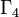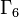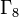# Element structure of groups of order 2^n

## Contents

This article gives specific information, namely, element structure, about a family of groups, namely: groups of order 2^n.
View element structure of group families | View other specific information about groups of order 2^n

This article describes the element structure of groups of order 2^n, i.e., groups whose order is a power of 2.$n$$2^n$ Number of groups of order$2^n$ Information on groups Information on element structure
0 1 1 trivial group --
1 2 1 cyclic group:Z2 element structure of cyclic group:Z2
2 4 2 groups of order 4 element structure of groups of order 4
3 8 5 groups of order 8 element structure of groups of order 8
4 16 14 groups of order 16 element structure of groups of order 16
5 32 51 groups of order 32 element structure of groups of order 32
6 64 267 groups of order 64 element structure of groups of order 64
7 128 2328 groups of order 128 element structure of groups of order 128
8 256 56092 groups of order 256 element structure of groups of order 256
9 512 10494213 groups of order 512 element structure of groups of order 512

## Conjugacy class sizes

FACTS TO CHECK AGAINST FOR CONJUGACY CLASS SIZES AND STRUCTURE:
Divisibility facts: size of conjugacy class divides order of group | size of conjugacy class divides index of center | size of conjugacy class equals index of centralizer
Bounding facts: size of conjugacy class is bounded by order of derived subgroup
Counting facts: number of conjugacy classes equals number of irreducible representations | class equation of a group
Number of size 1 conjugacy classes Number of size 2 conjugacy classes Number of size 4 conjugacy classes Number of size 8 conjugacy classes Total number of conjugacy classes Order of group Number of groups with these conjugacy class sizes Description of the groups
1 0 0 0 1 1 1 trivial group only
2 0 0 0 2 2 1 cyclic group:Z2 only
4 0 0 0 4 4 2 cyclic group:Z4 and Klein four-group
8 0 0 0 8 8 3 abelian groups of order 8: cyclic group:Z8, direct product of Z4 and Z2, elementary abelian group:E8
2 3 0 0 5 8 2 the non-abelian groups of order 8: dihedral group:D8 and quaternion group
16 0 0 0 16 16 5 The abelian groups of order 16: cyclic group:Z16, direct product of Z4 and Z4, direct product of Z4 and Z4, direct product of Z8 and Z2, direct product of Z4 and V4, elementary abelian group:E16
4 6 0 0 10 16 6 The groups of order 16, class exactly two, the Hall-Senior family$\Gamma_2$: SmallGroup(16,3), nontrivial semidirect product of Z4 and Z4, M16, direct product of D8 and Z2, direct product of Q8 and Z2, central product of D8 and Z4
2 3 2 0 7 16 3 The groups of order 16, class exactly three, the Hall-Senior family$\Gamma_3$: dihedral group:D16, semidihedral group:SD16, generalized quaternion group:Q16
32 0 0 0 32 32 7 All the abelian groups of order 32
8 12 0 0 20 32 15 The Hall-Senior family (up to isocliny)$\Gamma_2$
2 15 0 0 17 32 2 The extraspecial groups (Hall-Senior family$\Gamma_5$): inner holomorph of D8 and central product of D8 and Q8
4 6 4 0 14 32 19 The Hall-Senior families$\Gamma_3$ (ten groups, class three) and$\Gamma_4$ (nine groups, class two)
2 3 6 0 11 32 5 The Hall-Senior families$\Gamma_6$ and$\Gamma_7$
2 7 0 2 11 32 3 The maximal class groups (family$\Gamma_8$): dihedral group:D32, semidihedral group:SD32, generalized quaternion group:Q32

## 1-isomorphism

### All groupings

We say that two groups are 1-isomorphic groups if there exists a bijection between them that restricts to an isomorphism on all cyclic subgroups on both sides, i.e., there exists a 1-isomorphism between them. Below, we briefly describe the equivalence classes of groups of order$2^n$ up to 1-isomorphism:$n$ Order$2^n$ Total number of groups (up to isomorphism) Number of equivalence classes (up to 1-isomorphism) List of equivalence classes of size more than one More information
0 1 1 1 -- --
1 2 1 1 -- --
2 4 2 2 -- --
3 8 5 5 -- --
4 16 14 12 (direct product of Z8 and Z2, M16) and (direct product of Z4 and V4, central product of D8 and Z4) Element structure of groups of order 16#1-isomorphism
5 32 51 38 [SHOW MORE] Element structure of groups of order 32#1-isomorphism
6 64 267  ? Too long to list Element structure of groups of order 64#1-isomorphism

### Pairs of abelian and non-abelian based on explanation

We consider pairs of the form (abelian group, non-abelian group) that are 1-isomorphic. Note that since finite abelian groups with the same order statistics are isomorphic, the non-abelian member cannot be shared between pairs. However, the abelian group could be shared between multiple pairs, i.e., a single abelian group could be 1-isomorphic to multiple non-abelian groups.

The table below provides information on the number of such pairs that can be explained on the basis of various correspondences. As we go from left to right, the correspondence becomes progressively more generic, so the numbers in the later columns include the groups satisfying the stronger, more specific correspondences from earlier columns (the exception being the linear halving generalization of Lazard correspondence column, which is not more generic than its preceding column).$n$$2^n$ Number of 1-isomorphic (abelian, non-abelian) pairs Number of pairs arising via linear halving generalization of Baer correspondence, with intermediary class two Lie ring Number of pairs arising via cocycle halving generalization of Baer correspondence, with intermediary class two Lie cring Number of pairs arising via cocycle skew reversal generalization of Baer correspondence, with intermediary class two near-Lie cring Number of pairs arising via linear halving generalization of Lazard correspondence, with intermediary nilpotent Lie ring Number of pairs arising via cocycle halving generalization of Lazard correspondence Unexplained so far?
0 1 0 0 0 0 0 0 0
1 2 0 0 0 0 0 0 0
2 4 0 0 0 0 0 0 0
3 8 0 0 0 0 0 0 0
4 16 2 0 2 2 0 2 0
5 32 8 0 6 7 1 6 1
6 64 29 3 17 19 4 21 6
7 128 120  ? 50 55  ?  ?  ?